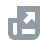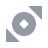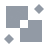Pay with
Markets
NFT
New
English
USDAccount FunctionsTutorialBinance Fan TokenBinance EarnCrypto Deposit/WithdrawalSpot & Margin TradingCrypto Derivatives
Futures Contracts
Introduction to Binance Futures
Multi-Assets Mode
USDⓈ-M Futures Contracts
COIN-M Futures Contracts
Futures Guide
Cross Collateral
Hedge Mode
Types of Orders
Binance Futures Welcome Bonus Coupon
Futures Service Agreement
Binance Futures Related Programs
Index
Portfolio Margin
Options
Leveraged TokensFinanceAPISecurityOther TopicsBinance ConvertNFTVIP

# How to Calculate Margin in Multi-Assets Mode

2022-07-06 07:02

## .css-6hm6tl{box-sizing:border-box;margin:0;min-width:0;color:#1E2329;}How to check your account margin ratio under the Multi-Assets Mode?

If you select the [Multi-Assets Mode], you will see a [Multi-Assets] tag on top of the Margin Ratio widget.
Since your margin balance is shared across multiple positions in the Multi-Assets Mode, please monitor your margin ratio closely. Once the margin ratio reaches 100%, all positions will be liquidated.

## How to calculate the margin ratio in the Multi-Assets Mode?

You can use the following equation to calculate your margin ratio in the Multi-Assets Mode:
Account Margin Ratio = Account Maintenance Margin / Account Equity
• Account Maintenance Margin: Sum of the Maintenance Margin of all cross positions (in USD).
• Account Equity: Sum of margin balance of all cross positions (in USD).
Please note that the margin ratio is based on the prevailing market rate for USDTUSD and BUSDUSD pairs.
Example:
Suppose the asset pairs and their respective floating conversion rates are as follows:
You want to trade two contracts, BTCUSDT perpetual and ETHBUSD_210326 with leverage of 100x and 50x respectively. In this scenario, the Maintenance Margin Ratio will be 0.8% and 1% respectively. Whether you are using the Isolated or the Cross Margin Mode, the account status and calculation changes of various parameters are as follows:

### 1. Initial state - No open positions

Under the Single-Asset Mode:
USDT available for order = 200 BUSD available for order = 220
Under the Multi-Assets Mode:
Account Equity = ∑min(assetEquity*bidRate, assetEquity*askRate) = 200 * 0.9801 + 220 * 1 = 416.02
As there are no open positions, assetCrossInitialMargin = 0
uniAvailableForOrder = accountEquity-∑(assetCrossInitialMargin*askRate) = 416.02 - 0 = 416.02
USDT Available for Order = 416.02 / 0.99495 = 418.13
BUSD Available for Order = 416.02 / 1 = 416.02
Account Maintenance Margin Ratio = 0
Margin Ratio = Account Maintenance Margin / Account Equity = 0

### 2. With open positions

Account Maintenance Margin = ∑assetMM*askRate = 0.5 * 20,000 * 0.008 * 0.99495 + 20 * 600 * 0.01 * 1 = 199.596
As there is no unrealized PnL, Account Equity = accountWalletBalance = 416.02
uniAvailableForOrder = Account Equity - ∑(assetCrossInitialMargin * askRate) = 416.02 - (0.5 * 20,000 * 0.01 * 0.99495 + 20 * 600 * 0.02 * 1)= 76.525
USDT Available for Order = 76.525 / 0.99495 = 76.91
BUSD Available for Order = 76.525 / 1 = 76.525
Margin Ratio = Account Maintenance Margin / Account Equity = 199.596 / 416.02 = 0.47977 = 47.98%

### 3. With open positions and unrealized PnL

Account Maintenance Margin = ∑assetMM*askRate = 0.5 * 19,000 * 0.008 * 0.99495 + 20 * 620 * 0.01 * 1 = 199.61
With unrealized PnL, Account Equity = ∑min(assetEquity*bidRate, assetEquity*askRate) = -300 * 0.99495 + 620 * 1 = 321.515
uniAvailableForOrder = Account Equity - ∑(assetCrossInitialMargin * askRate) = 321.515 - (0.5 * 19,000 * 0.01 * 0.99495 + 20 * 620 * 0.02 * 1) = -21
USDT Available for Order and BUSD Available for Order are both 0
Margin Ratio = Account Maintenance Margin / Account Equity = 199.61 / 321.515 = 0.62084 = 62.08%